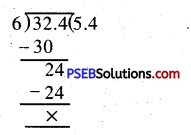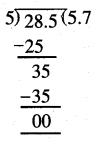# PSEB 5th Class Maths Solutions Chapter 6 Measurement Ex 6.5

Punjab State Board PSEB 5th Class Maths Book Solutions Chapter 6 Measurement Ex 6.5 Textbook Exercise Questions and Answers.

## PSEB Solutions for Class 5 Maths Chapter 6 Measurement Ex 6.5

Question 1.
The cost of 1 m cloth for pants is ₹ 265.50 and there is 24 m cloth in a roll. Find the cost of one bundle.
Solution:
Cost of 1 m cloth for pants = ₹ 265,50
Cost of 24 m cloth in a roll
= ₹ 265.50 × 24
= ₹ 6372.00
= ₹ 6372Question 2.
The weight of a box of mangoes is 32.4 kg. A shopkeeper wants to make 6 packets from this. How many kilograms of mangoes will be there in each packet?
Solution:
The weight of the box of mangoes = 32.4 kg
Number of packets = 6
Weight of mangoes in each box = 32.4 kg ÷ 6= 5.4 kg

Question 3.
A vessel contains 28.5 7 oil. It is poured into. 5 small containers. How much milk will be there in one small container?
Solution:
Quantity of milk in the given vessel = 28.5 l
Number of small containers = 5
Quantity of milk in each of small container = 28.5 ÷ 5= 5.7 l

Question 4.
1 bundle of copies weighs 9.8 kgs. Find the weight of 14 such bundles.
Solution:
Weight of 1 bundle of copies = 9.8 kg
Weight of 14 bundles of copies
= 9.8 × 14 kg
= 137.2 kg.Question 5.
The length of a stick is 12.7 cm. Find the length of 7 such sticks.
Solution:
The length of 1 stick = 12.7 cm
The length of 7 sticks = 12.7 × 7 cm
= 88.9 cm